# Exercise 11.2 Perimeter-and-Area -NCERT Solutions Class 7

Go back to  'Perimeter and Area'

## Chapter 11 Ex.11.2 Question 1

Find the area of each of the following parallelograms: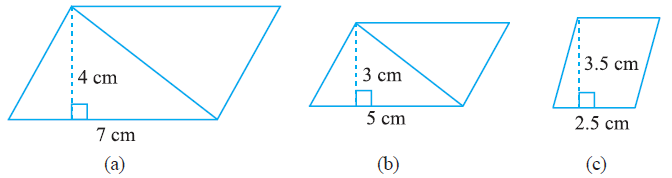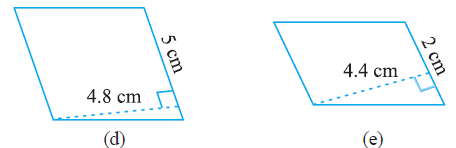### Solution

What is known?

Base and height of the parallelogram.

What is unknown?

Area of the parallelogram.

Reasoning:

Since base and height of the parallelogram is given, area of the parallelogram can be calculated by multiplying base with height.

Steps:

(a)

Given,

Base of parallelogram $$= 7 \,\rm cm$$

Height of parallelogram $$= 4 \,\rm cm$$

We know that,

\begin{align}&\text{Area of parallelogram}\\ &= {\rm{Base}} \times {\rm{Height}} \end{align}

\begin{align}&= 7 \times 4\\&= 28{\rm{ }}{\,\rm c{m^2}}\end{align}

(b)

Given,

Base of parallelogram $$= 5 \,\rm cm$$

Height of parallelogram $$= 3 \,\rm cm$$

\begin{align}& \text{Area of parallelogram} \\ &= {\rm{Base}} \times {\rm{Height}} \end{align}

\begin{align}&= 5 \times 3\\& = 15{\rm{ }}{\,\rm c{m^2}}\end{align}

(c)

Given,

Base of parallelogram $$= 2.5 \,\rm cm$$

Height of parallelogram $$= 3.5 \,\rm cm$$

\begin{align}& \text{Area of parallelogram} \\ &= {\rm{Base}} \times {\rm{Height}} \end{align}

\begin{align}&= 2.5 \times 3.5\\&= 8.75{\rm{ }}{\,\rm c{m^2}}\end{align}

(d)

Given

Base of parallelogram $$= 5 \,\rm cm$$

Height of parallelogram $$= 4.8 \,\rm cm$$

\begin{align}& \text{Area of parallelogram} \\ &= {\rm{Base}} \times {\rm{Height}} \end{align}

\begin{align}&= 5 \times 4.8\\&= 24{\rm{ }}{ \, \rm c{m^2}}\end{align}

(e)

Given

Base of parallelogram $$= 2 \,\rm cm$$

Height of parallelogram $$= 4.4 \,\rm cm$$

\begin{align}& \text{Area of parallelogram} \\ &= {\rm{Base}} \times {\rm{Height}} \end{align}

\begin{align}&= 2 \times 4.4\\&= 8.8{\rm{ }}{\,\rm c{m^2}}\end{align}

## Chapter 11 Ex.11.2 Question 2

Find the area of each of the following triangles: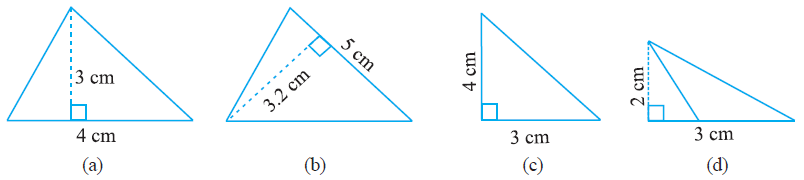### Solution

What is known?

Base and height of triangles.

What is the unknown?

The area of the triangles.

How can you use the known information to arrive at the solution?

Base and height of a triangle is already given. Area of triangle is equal to half of product of base and height.

Steps:

(a) Given,

Base $$= 3 \,\rm cm$$

Height $$= 4 \,\rm cm$$

We know that

\begin{align}&\text{Area of triangle} \\ &= \frac{1}{2} \times {\rm{Base}} \times {\rm{Height}} \end{align}

\begin{align}&= \frac{1}{2} \times {\rm{3}} \times {\rm{4}}\\&= {\rm{6}}\;{\rm{c}}{{\rm{m}}^2}\end{align}

(b) Given

Base $$= 5 \,\rm cm$$

Height $$= 3.2 \,\rm cm$$

\begin{align}&\text{Area of triangle} \\ &= \frac{1}{2} \times {\rm{Base}} \times {\rm{Height}} \end{align}

\begin{align} &= \frac{1}{2} \times {\rm{5}} \times {\rm{3}}{\rm{.2}}\\&= {\rm{8}}\;{\rm{c}}{{\rm{m}}^2}\end{align}

(c) Given

Base $$= 3 \,\rm cm$$

Height $$= 4 \,\rm cm$$

\begin{align}&\text{Area of triangle} \\ &= \frac{1}{2} \times {\rm{Base}} \times {\rm{Height}} \end{align}

\begin{align}&= \frac{1}{2} \times {\rm{3}} \times {\rm{4}}\\& = {\rm{6}}\;{\rm{c}}{{\rm{m}}^2}\end{align}

(d) Given

Base $$= 3 \,\rm cm$$

Height $$= 2 \,\rm cm$$

\begin{align}&\text{Area of triangle} \\ &= \frac{1}{2} \times {\rm{Base}} \times {\rm{Height}} \end{align}

\begin{align}& = \frac{1}{2} \times {\rm{3}} \times {\rm{2}}\\&= {\rm{3}}\;{\rm{c}}{{\rm{m}}^2}\end{align}

## Chapter 11 Ex.11.2 Question 3

Find the missing values:

 S. No. Base Height Area of Parallelogram a. $$20 \,\rm cm$$ $$246 \,\rm cm^2$$ b. $$15 \,\rm cm$$ $$154.5 ^\,\rm cm^2$$ c. $$8.4 \,\rm cm$$ $$48.72 \,\rm cm^2$$ d. $$15.6 \,\rm cm$$ $$16.38\,\rm cm^2$$

### Solution

What is known?

Area of the parallelogram.

What is unknown?

Base and height of the parallelogram.

Reasoning:

Area of all the parallelograms is given but in some parts of this question base and in some other parts height of the parallelogram is given. Put the values of the given part directly into the equation of area of parallelogram and find the unknown value.

Steps:

(a) Given,

Base of parallelogram $$= 20 \,\rm cm$$

Area of parallelogram $$= 246 \,\rm cm^2$$

Height of parallelogram $$= \,?$$

We know that,

\begin{align}&\text{Area of parallelogram}\\ &={\rm{Base}} \times {\rm{Height}}\end{align}

\begin{align}246 &= 20 \times {\rm{Height}}\\{\rm{Height}} &= \frac{{246}}{{20}}\\{\rm{Height}} &= 12.3\;\rm cm\end{align}

(b) Given

Height of parallelogram $$= 15\,\rm cm$$

Area of parallelogram $$= 154.5 \,\rm cm^2$$

Base of parallelogram $$= \,?$$

We know that,

\begin{align}&\text{Area of parallelogram}\\ &={\rm{Base}} \times {\rm{Height}}\end{align}

\begin{align}154.5 &= {\rm{Base}} \times {\rm{15}}\\{\rm{Base}} &= \frac{{154.5}}{{15}}\\{\rm{Base}} &= 10.3\;\rm cm\end{align}

(c) Given

Height of parallelogram $$= 8.4\,\rm cm$$

Area of parallelogram $$= 48.72 \,\rm cm^2$$

Base of parallelogram $$= \,?$$

We know that,

\begin{align}&\text{Area of parallelogram}\\ &={\rm{Base}} \times {\rm{Height}}\end{align}

\begin{align}48.72 &= {\rm{Base}} \times {\rm{8}}{\rm{.4}}\\{\rm{Base}} &= \frac{{48.72}}{{8.4}}\\{\rm{Base}} &= 5.8\;\rm cm\end{align}

(d) Given

Base of parallelogram $$= 15.6 \,\rm cm$$

Height of parallelogram $$= \,?$$

Area of parallelogram $$= 16.38 \,\rm cm^2$$

We know that,

\begin{align}&\text{Area of parallelogram}\\ &={\rm{Base}} \times {\rm{Height}}\end{align}

\begin{align} 16.38 &= {\rm{Base}} \times {\rm{15}}{\rm{.6}}\\{\rm{Base}} &= \frac{{16.38}}{{15.6}}\\{\rm{Base}} &= 1.05\;\rm cm\end{align}

## Chapter 11 Ex.11.2 Question 4

Find the missing values:

 S. No. Base Height Area of Triangle a. $$15 \,\rm cm$$ $$87 \,\rm cm^2$$ b. $$31.4 \,\rm mm$$ $$1256\,\rm mm^2$$ c. $$22 \,\rm cm$$ $$170.5 \,\rm cm^2$$

### Solution

What is known?

Area of triangle.

What is unknown?

Base and height of the triangle.

Reasoning:

In this question, area of the triangles are given. In some parts of the question base of the triangle and in some other parts height of the triangle is given. Put the values of the given part directly into the formula of area of triangle and find the unknown value.

Steps:

(a) Given,

Area of the triangle $$= 87 \,\rm cm^2$$

Base $$= 15 \,\rm cm$$

Height $$=\,?$$

We know that,

\begin{align}&{\text{Area of triangle}} \\ &= \frac{1}{2} \times {\rm{Base}} \times {\rm{Height}} \end{align}

\begin{align}87 &= \frac{1}{2} \times 15 \times {\rm{Height}}\\\\{\rm{Height}} &= \frac{{2 \times 87}}{{15}}\\\\{\rm{Height}} &= \frac{{174}}{{15}}\\\\{\rm{Height}} &= 11.6\;\rm{cm}\end{align}

(b) Given,

Area of the triangle $$= 1256\,\rm mm^2$$

Height$$= 31.4 \,\rm mm$$

Base $$=\,?$$

We know that,

\begin{align}&{\text{Area of triangle}} \\ &= \frac{1}{2} \times {\rm{Base}} \times {\rm{Height}} \end{align}

\begin{align}1256 &= \frac{1}{2} \times {\rm{Base}} \times {\rm{31}}{\rm{.4}}\\\\{\rm{Base}} &= \frac{{2 \times 1256}}{{31.4}}\;\\\\{\rm{Base}} &= \frac{{2512}}{{31.4}}\\\\{\rm{Base}} &= 80\; \rm mm\\\end{align}

(c) Given,

Area of the triangle $$= 170.5 \,\rm cm^2$$

Base $$= 22 \,\rm cm$$

Height $$=\,?$$

We know that,

\begin{align}&{\text{Area of triangle}} \\ &= \frac{1}{2} \times {\rm{Base}} \times {\rm{Height}} \end{align}

\begin{align}170.5 &= \frac{1}{2} \times {\rm{22}} \times {\rm{Height}}\\{\rm{Height}} &= \frac{{2 \times 170.5}}{{22}}\\{\rm{Height}} &= \frac{{341}}{{22}}\\{\rm{Height}} &= 15.5\; \rm cm\end{align}

## Chapter 11 Ex.11.2 Question 5

$$PQRS$$ is a parallelogram (Fig 11.23). $$QM$$ is the height from $$\, Q \,$$ to $$SR$$ and $$QN$$ is the height from$$\, Q \,$$ to $$PS$$. If $$SR$$ $$= 12\,\rm cm$$ and $$QM$$ $$= 7.6 \,\rm cm$$.

Find:

(a) the area of the parallelogram $$PQRS$$

(b) $$QN$$, if $$PS$$ $$= 8 \,\rm cm$$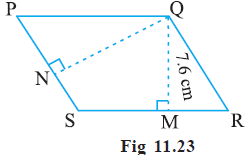### Solution

What is known?

$$PQRS$$ is a parallelogram. $$QM$$ is the height from $$\, Q \,$$ to $$SR$$ and $$QN$$ is the height from $$\, Q \,$$ to $$PS$$. Also, $$SR$$ $$= 12\,\rm cm$$ and $$QM$$ $$= 7.6 \,\rm cm$$.

What is unknown?

(a) The area of the parallelogram $$PQRS$$ and (b) $$QN$$, if $$PS$$ $$= 8 \,\rm cm$$

Reasoning:

First, using the given information (base $$SR$$ = 12cm and perpendicular height $$QM$$ = 7.6cm), find out the area of parallelogram $$PQRS$$. Now, area of $$PQRS$$ and base $$PS$$ is known. Again, by using the formula of area of parallelogram, find out height $$QN$$.

Steps:

(a) Given, $$SR$$ $$= 12\,\rm cm$$ and

$$QM$$ $$= 7.6 \,\rm cm$$.

\begin{align} & \text{Area of parallelogram} \; PQRS \\ &= {\rm{Base}} \times {\rm{Height}} \end{align}

\begin{align}&= SR \times QM \\&= 12 \times 7.6\\&= 91.2 \, \rm cm^2\end{align}

(b) Given, Base $$PS$$ $$= 8 \,\rm cm$$

Area of the parallelogram $$= 91.2 \rm\, cm^2$$ [calculated in part (a)]

\begin{align} & \text{Area of parallelogram}\; PQRS \\ &= {\rm{Base}} \times {\rm{Height}} \end{align}

\begin{align} 91.2 &= 8 \times QN \\{{QN}} &= \frac{91.2}{8}\\{{QN}} &= 11.4\;\rm cm\end{align}

## Chapter 11 Ex.11.2 Question 6

$$DL$$ and $$BM$$ are the heights on sides $$AB$$ and $$AD$$ respectively of parallelogram $$ABCD$$ (See the below figure). If the area of the parallelogram is $$1470 \,\rm cm^2$$, $$AB$$$$= 35 \,\rm cm$$ and $$AD$$ = 49 cm, find the length of $$BM$$ and $$DL$$.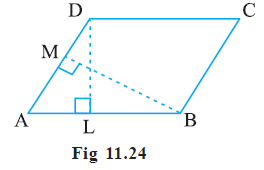### Solution

What is known?

The area of the parallelogram and its two sides, $$AB$$ and $$AD$$. $$DL$$ and $$BM$$ are the heights on sides $$AB$$ and $$AD$$ respectively of parallelogram $$ABCD$$.

What is unknown?

The length of the perpendiculars $$DL$$ and $$BM$$ to sides $$AB$$ and $$AD$$.

Reasoning:

$$AB \,$$is the base of the parallelogram and the perpendicular on $$AB \,$$is $$DL$$ (the height). The area of parallelogram is given as $$1470 \,\rm cm^2.$$ By using the formula of area of parallelogram we can find the height $$DL$$. Similarly, if you take $$AD$$ as the base and $$BM$$ as perpendicular (height) on $$AD$$, again by using the formula of area of parallelogram we can find the length of $$BM$$.

Steps:

Given, $$AB$$$$= 35 \,\rm cm$$, $$BC = 49 \rm \,cm$$ and Area $$= 1470 \,\rm cm^2$$

Area of parallelogram ABCD $$=$$  Base $$(AB)$$ $$\times$$ Height $$(DL)$$

\begin{align}1470 &= 35 \times {{DL}}\\{{DL}} &= \frac{{1470}}{{35}}\\{{DL}} &= 42\;\rm cm\end{align}

Area of parallelogram ABCD  $$=$$  Base $$(BC)$$ $$\times$$ Height $$(BM)$$

\begin{align}1470 &= 49 \times {{BM}}\\{{BM}} &= \frac{{1470}}{{49}}\\{{BM}} &= 30\;\rm cm\end{align}

## Chapter 11 Ex.11.2 Question 7

$$\triangle ABC$$ is right angled at$$\, A \,$$ (Fig 11.25).  $$AD$$ is perpendicular to $$BC$$. If $$AB = 5 \,\rm cm$$, $$BC = 13 \,\rm cm$$ and $$AC = 12 \,\rm cm$$, Find the area of $$\triangle ABC$$. Also find the length of $$AD$$.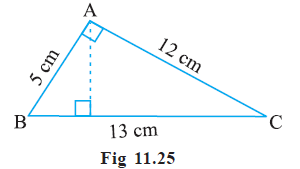### Solution

What is known?

$$\triangle ABC$$ is right angled at $$A$$, $$AD$$ is perpendicular to side $$BC$$. Length of three sides of the triangle is also given.

What is unknown?

The area of $$\triangle ABC$$ and the length of perpendicular $$AD$$.

Reasoning:

First, find the area of triangle using base $$(5 \,\rm cm)$$ and height $$(12 \,\rm cm)$$. Now, the area of the triangle is known, and the base is given as $$BC = 13 \,\rm cm$$ and you must find the height $$AD$$ by using the formula of area of triangle.

Steps:

In right angled $$\triangle BAC$$,

$$AB = 5 \,\rm cm$$, $$BC = 13 \,\rm cm$$ and $$AC = 12 \,\rm cm$$.

Area of triangle $$BAC$$  $$=$$ $$\frac{1}{2}$$ Base  $$\times$$ Height

\begin{align}&= \frac{1}{2} \times 5 \times 12\\&= 30\;\rm{c{m^2}}\end{align}

Area of triangle $$ABC$$  $$=$$ $$\frac{1}{2}$$ Base  $$\times$$ Height

\begin{align}30 &= \frac{1}{2} \times 13 \times {\rm{AD}}\\{\rm{AD}} &= \frac{{30 \times 2}}{{13}}\\{\rm{AD}} &= \frac{{60}}{{13}}\\{\rm{AD}} &= 4.61\;cm\end{align}

## Chapter 11 Ex.11.2 Question 8

$$\triangle ABC$$ is isosceles with $$AB = AC = 7.5 \,\rm cm$$ and $$BC = 9 \,\rm cm$$ (Fig 11.26). The height $$AD$$ from $$A$$ to $$BC$$, is $$6 \,\rm cm$$. Find the area of $$\triangle ABC$$. What will be the height from $$C$$ to $$AB$$ i.e., $$CE\,?$$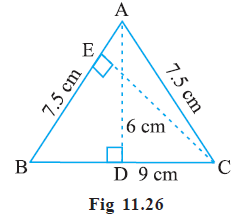### Solution

What is known?

$$\triangle ABC$$ is isosceles with $$AB = AC = 7.5 \,\rm cm$$ and $$BC = 9 \,\rm cm$$. The height $$AD$$ from $$A$$ to $$BC,$$ is $$6 \,\rm cm.$$

What is unknown?

The area of $$\triangle ABC$$ and the height from $$C$$ to $$AB$$ i.e., $$CE.$$

Reasoning:

First, find the area of the given triangle using base as $$9 \,\rm cm$$ and height as $$6\,\rm cm.$$ Now, the area of triangle is known, take $$AB = 7.5 \,\rm cm$$ as the base and find height $$CE$$ using the formula of area of triangle.

Steps:

In $$\triangle ABC$$, $$AD = 6 \,\rm cm$$ and $$BC = 9\,\rm cm$$

Area of triangle $$ABC$$  $$=$$ $$\frac{1}{2}$$ Base  $$\times$$ Height

\begin{align}& = \frac{1}{2} \times {\rm{BC}} \times {\rm{AD}}\\&= \frac{1}{2} \times 9 \times 6\\&=27\;c{m^2}\end{align}

Now,

Area of triangle $$ABC$$  $$=$$ $$\frac{1}{2}$$ $$AB$$  $$\times$$ $$CE$$

\begin{align}{\rm{27}} &= \frac{1}{2} \times {\rm{7}}{\rm{.5}} \times {\rm{CE}}\\{\rm{CE}} &= \frac{{2 \times {\rm{27}}}}{{{\rm{7}}{\rm{.5}}}}\\{\rm{CE}} &= \frac{{54}}{{{\rm{7}}{\rm{.5}}}}\\{\rm{CE}} &= 7.2\;\rm{cm}\end{align}

Related Sections
Related Sections
Instant doubt clearing with Cuemath Advanced Math Program